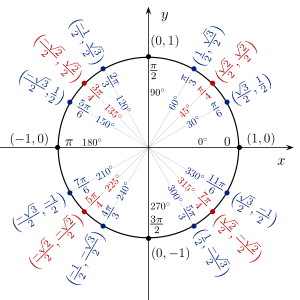# Movement Vector

How would I make it so when a part is moving diagonally its movement vector is (0.5,0,0.5) instead of (0.7,0,0.7)?

This is what I mean by the movement vector:

`rootPart.CFrame:vectorToObjectSpace(rootPart.Velocity / humanoid.WalkSpeed)`

When moving forward this would print (0,0,1) and when moving diagonally it would print (0.7,0,0.7).sqrt(2)/2 is ~0.7

1 Like

Why would you want to move at `(0.5,0,0.5)` instead of `(0.7,0,0.7)`? Assuming that you want to move at one stud per second, the speed of the first one is `sqrt(0.5^2 + 0.5^2) ~~ 0.7071` while the second one is `sqrt(0.7^2 + 0.7^2) ~~ 0.9899`

I do not want the player to move at that speed, I only want the movement vector to be (0.5,0,0.5) diagonally because I’m using it to blend 4 directional animations (Forward, Backward, Left and Right) by setting their weight to the direction. The problem I’m having is that when the player is moving diagonally, two animations have a weight of 0.7 which makes the diagonal animation look barely different from the sideways animation, if they were both at 0.5 it would look much better.

Right. The way I did it was divide the vector by the sum of its components:

`(0.7, 0, 0.7) / (0.7 + 0.7) = (0.7, 0, 0.7)/1.4 = (0.5, 0, 0.5)`

This makes sure that the sum of the weights is always 1.

Oh, and word of caution since you’re blending animations: Adjusting the weight of an animation to 0 will stop it (as in you’ll have to :Play() it again). Just watch out for this.

EDIT: Oh, and also be careful of division by zero when the player stops.

1 Like

How would I use your formula correctly?

I just did this and it does make the vector (0.5,0,0.5) when moving diagonally, but it also makes it so when moving completely forward instead of it being (0,0,1) it is (0,0,0.7).

rootPart.CFrame:vectorToObjectSpace((rootPart.Velocity/Humanoid.WalkSpeed)/1.4)

By components, I mean its x-, y-, and z-values. Don’t just use 1.4, that was just the example for (0.7, 0, 0.7)!

For `(0, 0, 1)`, the sum of its components would be `0 + 0 + 1 = 1`, and the resulting vector should be the same: `(0, 0, 1)/1 = (0, 0, 1)`

EDIT: Since you brought up negative values, get the sum of the absolute values of each component, and then divide the original vector by that sum instead.

1 Like

Basically you want this?

``````function someName(vector)
local n = (math.abs(vector.X)+math.abs(vector.Y)+math.abs(vector.Z))
return vector / (n > 0 and n or 1)
end``````
1 Like

You simply sum the components of the movement vector and then determine the relative in each direction.

Example:
(0.8, 0, .6)

Sum is 1.4 in absolute (in case of negatives)

Relative X component is .8 / 1.4 = .57
Relative Z component is .6 / 1.4 = .43

Positive/negative result in each component determine whether you should blend forward/backward or left/right, while its absolute value determines the blending factor of the animation.

4 Likes

Yes, this works fine, but the vector can only be 1, 0.5 or 0, for example even if the player moves forward slightly your function sets vector.Z to 1 immediately instead of 0.1 or whatever the actual speed of the player is. Is there any way to fix this without lerping/tweening?

@buildthomas yeah, but the problem with that is if the vector is (0,0,0.2) and I divide by the sum, it returns 1 instead of 0.2, giving the problem described above.

This is impossible, since MoveDirection works on the unit circle, we can prove that using the following:

Let x = 0, y = 0.2

x^2 + y^2 = 1
0.2^2 = 1?

That’s incorrect, so (0, 0.2) does not lie on the unit circle.

Now that we got that out of the way, using the method @buildthomas mentioned.

``````local notMoving = Vector3.new()

local function unitCircleRatio(v3)

if v3 == notMoving then
return notMoving
end

local x = math.abs(v3.X)
local z = math.abs(v3.Z)

local sum = (x + z)
return Vector3.new(x/sum, 0, z/sum)
end

local humanoid = script.Parent:WaitForChild("Humanoid")

while wait() do
print(unitCircleRatio(humanoid.MoveDirection))
end
``````

What I’m trying to say is that when the player moves even a little bit, with your function it snaps the vector to 1 immediately, but without it it doesn’t do that. I want to know if there is another formula to make the speed go up incrementally instead of snapping to 1 or 0.

As you can see it does not snap to -1 immediately when the player moves forward, but also has steps inbetween.

(sorry for the low quality, giant video, it was made with gyazo gif)

1 Like

Then you multiply the weights by the length of the movement vector. Sorry for assuming that was obvious. (Assuming the move vector increases/decreases smoothly)

1 Like

I assume by length you mean .Magnitude and the movement vector is the one before it is passed into the unitCircleRatio() function in @Darkmist101 post. If so then that defeats the purpose of me doing this calculation in the first place since multiplying it by the length of the original movement vector reverses the calculation and multiplying by the length of the new movement vector does nothing.

Dividing by the sum of components is not the inverse operation to multiplying by the vector length.

1 Like

If I multiply the weights (0.5 when moving diagonally) by the old movement vector length (1.4 when moving diagonally) it makes the weights 0.7 when moving diagonally which is exactly what I don’t want

EDIT:

Nevermind, disregard this.
I realised I could just clamp the length to 1, sorry and thanks to everyone who tried to help me out. Guess I’m just having a bad day today, mind is not working.